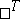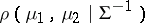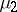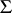# Mahalanobis distance

(diff) ← Older revision | Latest revision (diff) | Newer revision → (diff)

The quantitywhereare vectors andis a matrix (anddenotes transposition). The Mahalanobis distance is used in multi-dimensional statistical analysis; in particular, for testing hypotheses and the classification of observations. It was introduced by P. Mahalanobis , who used the quantityas a distance between two normal distributions with expectationsandand common covariance matrix. The Mahalanobis distance between two samples (from distributions with identical covariance matrices), or between a sample and a distribution, is defined by replacing the corresponding theoretical moments by sampling moments. As an estimate of the Mahalanobis distance between two distributions one uses the Mahalanobis distance between the samples extracted from these distributions or, in the case  where a linear discriminant function is utilized — the statistic, whereandare the frequencies of correct classification in the first and the second collection, respectively, andis the normal distribution function with expectation 0 and variance 1.

How to Cite This Entry:
Mahalanobis distance. Encyclopedia of Mathematics. URL: http://encyclopediaofmath.org/index.php?title=Mahalanobis_distance&oldid=17720
This article was adapted from an original article by A.I. Orlov (originator), which appeared in Encyclopedia of Mathematics - ISBN 1402006098. See original article